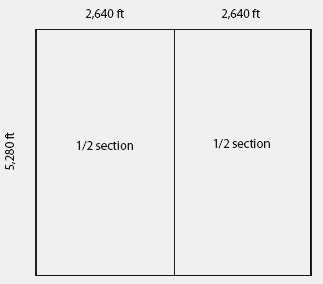half section

Also found in: Dictionary, Encyclopedia.

half sectionSee public land survey system for a full explanation.

Example: A section of land is 1 square mile, being 5,280 feet on each side and containing 640 acres. A half section can be the north or south half, or it can be the east or west half, but it can't be an arbitrary 320 acres somewhere in the middle. A half section would have one side of 5,280 feet and the other side of 2,640 feet.

Mentioned in ?
References in periodicals archive ?
The characteristic impedance of loss-less transmission line is proportional to square root of the ratio of inductance and capacitance, following this premise the input impedance of ACD full section should be half of the ACD half section because capacitance increased twice and inductance reduced to half compared to ACD half section.
A save and solve button at the bottom of the project editor initiates CFD calculations on the half section of the die exit.
Axially symmetric extrusion process allowed to carry out simulations in a half sections what reduced time of calculation.
To find the fractions represented by the arrows A, C, D, students need to divide the half sections into two equal parts, thus quarters, and correctly identify the number of quarters that comprises the quantity.
Since a single arch uses two half sections, I built a jig on which to construct a half.
She then used her drawing to show that every one and a half sections (see shaded part of small rectangle in figure 2) was equivalent to one spool of thread, and thus Jack had 5 spools of thread and some extra.
These stubs are clipped to the chassis rails with half sections of 1-1/4" conduit and hose clamps.
These transmission lines can be terminated in appropriate m-derived half sections and resistors equal to the characteristic impedance of the lines to minimize this effect.

Site: Follow: Share:
Open / Close